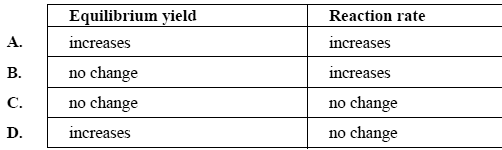Equilibrium exercise (2005 VCE) Sulfur dioxide and oxygen are mixed to form sulfur trioxide according to 2SO2(g) + O2(g) => 2SO3(g) Which one of the following best describes the effect of adding the catalyst V2O5 to the mixture?SolutionDeuterium (symbol D) is an isotope of hydrogen. Water made from deuterium has the symbol D2O and has similar properties to normal water. D2O ionises according to the equilibrium D2O => D+ + OD- Kd = [D+] [OD-] = 1.82 X 1016 M2 at 25°C. In a neutral solution of pure D2O at 25°C the concentration of D+, in mole per litre, is A. 1.00 X 107 B. 1.35 X 108 C. 0.91 X 1016 D. 1.82 X 1016 SolutionDinitrogen tetroxide (N2O4) is a colourless gas. It exists in equilibrium with nitrogen dioxide (NO2), a brown gas. The concentration of NO2 in for the reaction is N2O4(g) => 2NO2(g); K = 5.5 X 103 M at 25°C ....................................(1) Write the expression for the equilibrium constant for this reaction. Solution Some pure NO2 is placed in a gas syringe at 25°C and allowed to reach equilibrium. Keeping the volume constant the temperature is then raised to 35°C. The brown colour then becomes more intense. Is the above reaction (1) exothermic or endothermic? Explain your answer. Solution Keeping the temperature at 35°C the plunger of the syringe is then pushed in so as to halve the volume. Equilibrium is then re-established. Is the brown colour of the mixture more intense or less intense than before the volume was halved? SolutionGive the numerical value at 25°C of the equilibrium constant of the reaction below. NO2(g) =>1/ 2N2O2(g) SolutionTo live, the human body needs a regular supply of oxygen, which is distributed throughout the body by the red pigment, haemoglobin (Hb). Hb is carried around the body by the red blood cells in the blood. a. Write a simple equation showing oxygen reacting with haemoglobin. Solution b. Using this equation explain, in terms of equilibrium principles, how a low oxygen concentration can lead to the cells in a human body being deprived of oxygen. Solution c. At high altitudes, the pressure of atmospheric oxygen is significantly less than it is at sea level. People who live most of their lives on very high mountains normally have a number of special adaptations to living at high altitudes. One such adaptation is the possession of a signiÞ cantly higher red blood count (that is, a larger number of red blood cells in the blood) compared with people living at sea level. Explain how a high blood count is a useful adaptation to high altitude living. SolutionEthanoic acid (CH3COOH) is a weak acid in water. Write an equation showing the ionisation of ethanoic acid in water. Solution A 0.100 M solution of ethanoic acid in water at 25°C has a pH of 2.88. i. Calculate the hydrogen ion concentration in a 0.100 M solution of ethanoic acid. Solution ii. Calculate the acidity constant of ethanoic acid at 25°C. Solution At 25°C, methanoic acid (HCOOH) has an acidity constant that is approximately ten times greater than the acidity constant of ethanoic acid. i. Comparing two 0.10 M solutions of methanoic and ethanoic acids, which solution would have the higher pH? Give a simple qualitative explanation for your answer. Solution ii. Equal volumes of both solutions were titrated against a 0.10 M solution of NaOH. Which of the solutions, if either, would require the greater volume of the NaOH solution for complete neutralisation? Explain your conclusion. Solution